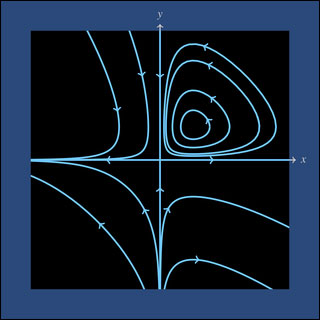18.03SC | Fall 2011 | Undergraduate

# Differential Equations

## Unit IV: First-order Systems

« Previous | Next »Nonlinear phase portrait. Modeled on the MIT mathlet Vector Fields.

In this unit we study systems of differential equations. A system of ODE’s means a DE with one independent variable but more than one dependent variable, for example:

x’ = x + y, y’ = _x_2 - y - t

is a 2x2 system of DE’s for the two functions x = x(t) and y = y(t).

As usual, we start with the linear case. Even for linear systems, though, it turns out that efficient solution methods require some new techniques, namely the machinery of matrix-vector algebra. A small investment in this background material yields an excellent return, giving both the linear theory in the general case and also the explicit computational methods for the solutions in the constant-coefficient case.

We finish this unit by showing some of the qualitative theory of DE’s for systems, linear and non-linear. Qualitative theory means finding out information about the solutions directly from the DE without actually having to solve it. We start with the linear case, and then show how we can use the results for linear constant-coefficient systems to gain information about certain non-linear systems using a technique called linearization.

« Previous | Next »

## Course Info

Fall 2011
##### Learning Resource Types
Lecture Videos
Recitation Videos
Exams with Solutions
Simulations
Lecture Notes
Problem Sets with Solutions